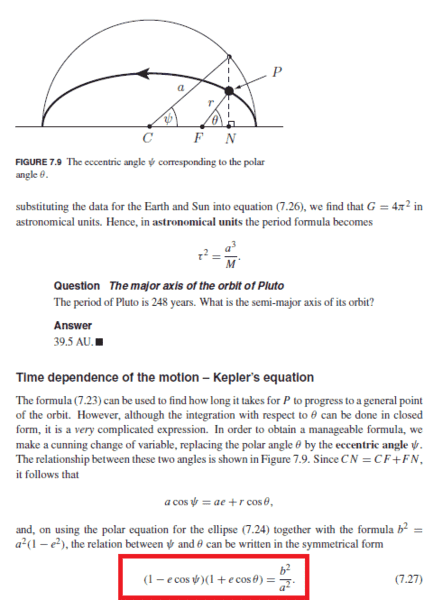# Question about the Kepler equation

## Homework Statement## Homework Equations

1/r = a/b2 * (1+e cosθ)

## The Attempt at a Solution

How do you derive 7.27?
I tried to substitute r in the orbit equation by (acosψ - ae) / cosθ and got

#### Attachments

TSny
Homework Helper
Gold Member
(cos ψ - e)/cosθ*(1+e cosθ)=b^2/a^2
I believe this is OK. You just need to manipulate it to get (7.27). I don't know the quickest way, but I found something that works. I don't want to give too much away.
Rearrange your equation as (cos ψ - e)*(1+e cosθ)=(b^2/a^2)*cosθ. Substitute for b^2/a^2 in terms of e. Multiply everything out and see if you can get to the result.

•Clara Chung
I tried hard by substituting (b^2/a^2) but still can't figure out the equation... Then I changed my mind to substitute for e. Thank you so much.
(cos ψ - e)*(1+e cosθ)=(b^2/a^2)*cosθ

(ecos ψ - 1+b^2/a^2)*(1+e cosθ)=(b^2/a^2)*cosθ*e

-(1-ecosψ)(1+ecosθ)+(b^2/a^2)e cosθ+(b^2/a^2)=(b^2/a^2)*cosθ*e

(1-ecosψ)(1+ecosθ)=(b^2/a^2)

•TSny
TSny
Homework Helper
Gold Member
Nice.